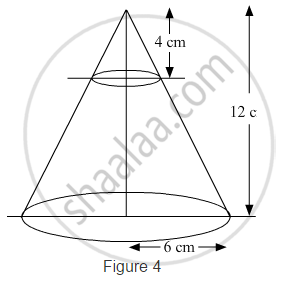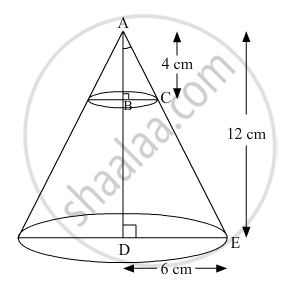# In Fig. 4, from the top of a solid cone of height 12 cm and base radius 6 cm, a cone of height 4 cm is removed by a plane parallel to the base. Find the total surface area of the remaining solid. - Mathematics

In Fig. 4, from the top of a solid cone of height 12 cm and base radius 6 cm, a cone of height 4 cm is removed by a plane parallel to the base. Find the total surface area of the remaining solid. (Use pi=22/7 and sqrt5=2.236)#### Solution

The remaining solid is a frustum of the given cone

Total surface area of the frustum = πl(r1+r2)+πr12+πr22

Where

h = Height of the frustum = 124 = 8 cm

r1 = Larger radius of the frustum = 6 cm

r2 = Smaller radius of the frustum

l = Slant height of the frustumIn the given figure, ∆ABC ​~ ∆ADE by AA similarity criterion.

:.(BC)/(DC)=(AB)/(AD)

=>r_2/6=4/12

r2=2 cm

We know

l = sqrt(h^2+(r_1-r_2)^2)

=>l=sqrt(8^2+(6-1)^2)

=>l = 4sqrt5

Total surface area of the frustum = πl(r1+r2)+πr12+πr22

= π×4sqrt5(6+2)+π×62+π×22

=pi(32sqrt5+40)

=22/7xx111.552

= 350.592 cm2

Hence, the total surface area of the remaining solid is 350.592 cm2.

Concept: Surface Area of a Combination of Solids
Is there an error in this question or solution?
Chapter 14: Surface Areas and Volumes - Exercise 14.3 [Page 79]

#### APPEARS IN

RD Sharma Class 10 Maths
Chapter 14 Surface Areas and Volumes
Exercise 14.3 | Q 20 | Page 79

Share